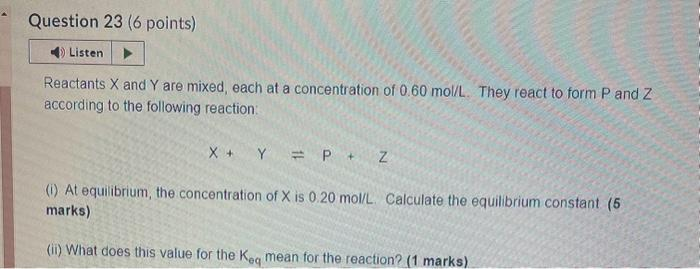# Question Solved1 Answerquestion 23 Reactants $$X$$ and $$Y$$ are mixed, each at a concentration of $$0.60$$ mol/L. They react to form $$P$$ and $$Z$$ according to the following reaction: $X+Y \Rightarrow P+Z$ (i) At equilibrium, the concentration of $$X$$ is $$0.20 \mathrm{~mol} / \mathrm{L}$$. Calculate the equilibrium constant (5 marks) (ii) What does this value for the $$\mathrm{K}_{\text {eq }}$$ mean for the reaction? (1 marks)question 23Transcribed Image Text: Reactants $$X$$ and $$Y$$ are mixed, each at a concentration of $$0.60$$ mol/L. They react to form $$P$$ and $$Z$$ according to the following reaction: $X+Y \Rightarrow P+Z$ (i) At equilibrium, the concentration of $$X$$ is $$0.20 \mathrm{~mol} / \mathrm{L}$$. Calculate the equilibrium constant (5 marks) (ii) What does this value for the $$\mathrm{K}_{\text {eq }}$$ mean for the reaction? (1 marks)
More
Transcribed Image Text: Reactants $$X$$ and $$Y$$ are mixed, each at a concentration of $$0.60$$ mol/L. They react to form $$P$$ and $$Z$$ according to the following reaction: $X+Y \Rightarrow P+Z$ (i) At equilibrium, the concentration of $$X$$ is $$0.20 \mathrm{~mol} / \mathrm{L}$$. Calculate the equilibrium constant (5 marks) (ii) What does this value for the $$\mathrm{K}_{\text {eq }}$$ mean for the reaction? (1 marks)